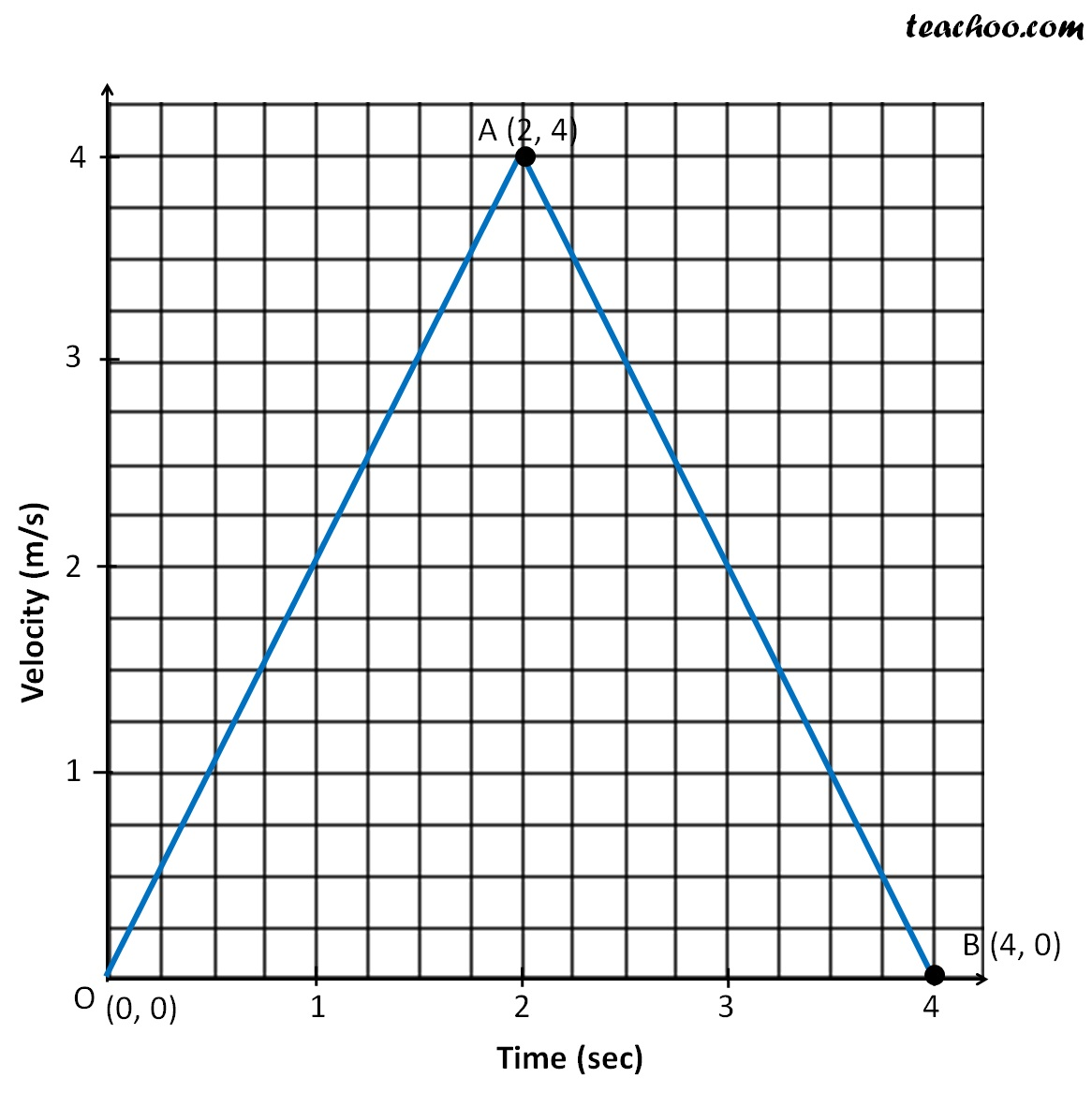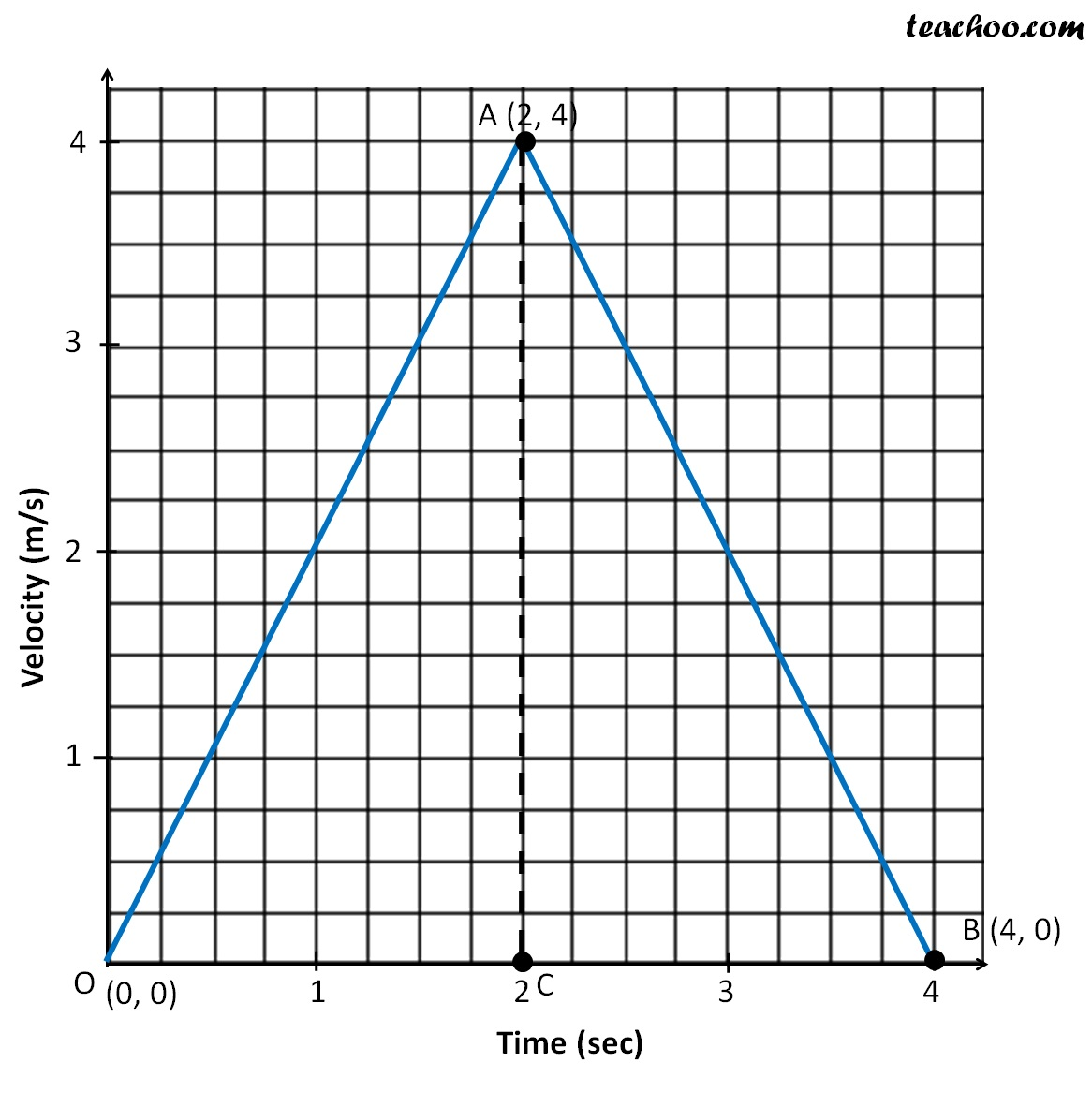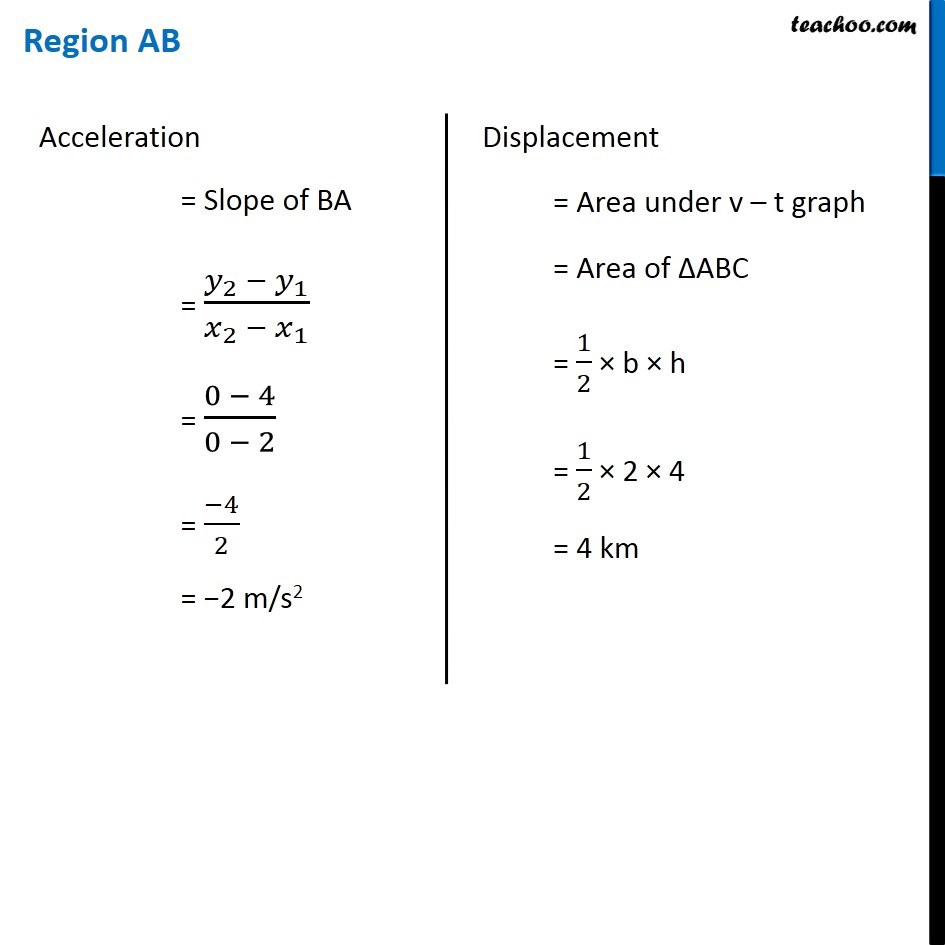## Find Displacement and Acceleration in region OA and OB. Also tell the type of motion in both the regions. And find the total displacementMarking point C in the graphMotion in region OA = Uniformly accelerated

Motion in region OB = Uniformly retarded

Total Displacement = Area of ∆ABO

= Area of ∆OAC + Area of ∆ABC

= 4m + 4m

= 8m

1. Class 9
2. Chapter 8 Class 9 - Motion
3. Extra Questions

Transcript

Region OA Acceleration = Slope of OA = (𝑦_2 − 𝑦_1)/(𝑥_2 − 𝑥_1 ) = (4 − 0)/(2 − 0) = 4/2 = 2 m/s2 Displacement = Area under v – t graph = Area of ∆OAC = 1/2 × b × h = 1/2 × 2 × 4 = 4 m Region AB Acceleration = Slope of BA = (𝑦_2 − 𝑦_1)/(𝑥_2 − 𝑥_1 ) = (0 − 4)/(0 − 2) = (−4)/2 = −2 m/s2 Displacement = Area under v – t graph = Area of ∆ABC = 1/2 × b × h = 1/2 × 2 × 4 = 4 km

Extra Questions

Class 9
Chapter 8 Class 9 - Motion